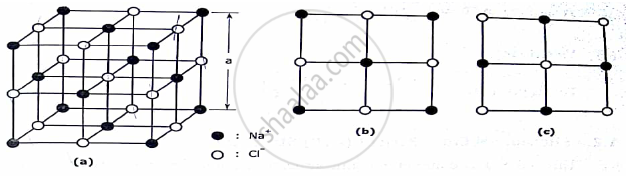# Draw and explain the unit cell of sodium chloride (NaCl) crystal determine effective number of NaCl molecule per unit cell and co-ordination number. - Applied Physics 1

Draw and explain the unit cell of sodium chloride (NaCl) crystal determine effective number of NaCl molecule per unit cell and co-ordination number.

#### Solution

NaCl STRUCTURE:-

This is an ionic structure in which the Na+ions and Cl
ions are alternately arranged. It is a combination of two FCC sublattice one made up of Na+ions and the other of Cl sublattice is translated through the other along the cube edges. ions as if one

NaCl unit cell with Na+ ions occupying the regular FCC lattice points with Cl
alternate points. A face of this unit cell is shown. ions positioned at Another NaCl unit cell can be considered with the positions of Na+ and The face of such a unit cell is shown.

NaCl UNIT CELL PARAMETER:(A) Total number of molecule / unit cells
Calculation for Na = Here Na forms a FCC structure. Hence total number of Na+ ions = 4

Calculation for Cl = There are 12Cl ions at the edges. Every edge lattice points is shared by four neighbouring unit cell. Hence every edge lattice point carries ¼ of an atom. There is one whole Cl ion at the centre of the structure. Hence ,

Total number of Cl ions = (12 ×1/4) + 1 = 4.

Since there are 4 Na ions and four Cl present in a unit cell. ions in a NaCl unit cell , there are four NaCl moleculeHence number of molecule / unit cell = 4.

Since NaCl is an ionic structure and cations are smaller than anions it is assumed that radius of cation = rc and the radius of an anion = rA .

(c) Atomic packing factor(APF)

APF = ((4 xx 4/3 πr^3_c) xx (4 xx 4/3πr^3_A))/a^3  it is found that a = 2rc + 2rA

Hence ,   APF=( (2π)/3) (r_c^3 + r_A^3)/((rC+rA)^3)

(d) Void space.
This is given by [1-((2π)/3) (r_c^3 +r_A^3) /(rC+rA)^3]

Concept: Study of characteristics of unit cell of Diamond, ZnS, NaCl and HCP;
Is there an error in this question or solution?
2016-2017 (December) CBCGS

Share